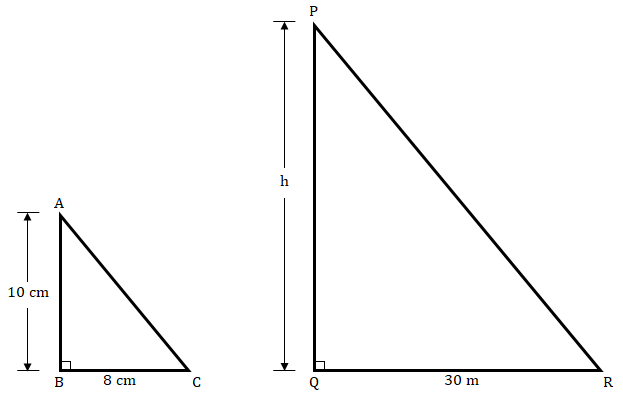"
">

# A vertical stick 10 cm long casts a shadow 8 cm long. At the same time, a tower casts a shadow 30 m long. Determine the height of the tower."

Given:

A vertical stick 10 cm long casts a shadow 8 cm long.

A tower casts a shadow 30 m long.

To do:

We have to find the height of the tower.

Solution:

Length of the stick $= 10\ cm$.

Length of the stick’s shadow $= 8\ cm$.

Length of the tower’s shadow $= 30\ m = 30\times100\ cm=3000\ cm$.

In $ΔABC$ and $ΔPQR$

$\angle ABC = \angle PQR = 90^o$

$\angle ACB = \angle PRQ$   (Angular elevation of Sun is same)

Therefore,

$ΔABC ∼ ΔPQR$    (By AA similarity)

This implies,

$\frac{AB}{BC} = \frac{PQ}{QR}$    (Corresponding sides are proportional)

$\frac{10}{8} = \frac{PQ}{3000}$

$PQ = \frac{3000\times10}{8}$

$PQ = \frac{15000}{4}$

$PQ = 3750\ cm$

$PQ=\frac{3750}{100}\ m$

The height of the tower(PQ) is $37.5\ m$.

Updated on: 10-Oct-2022

224 Views